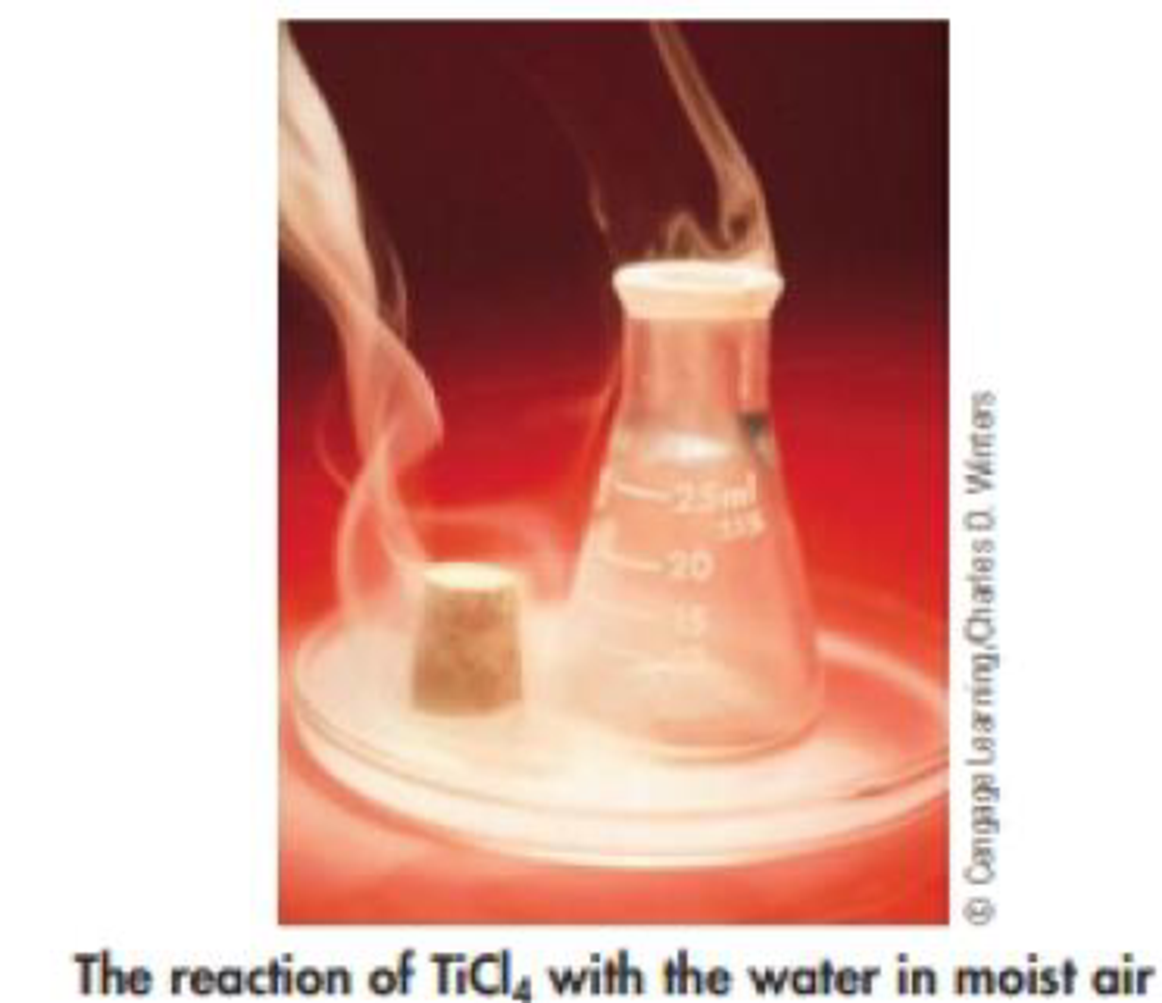# Some metal halides react with water to produce the metal oxide and the appropriate hydrogen halide (see photo). For example, TiCl 4 (l) + 2 H 2 O(l) → TiO 2 (s) + 4 HCl(g) (a) Name the four compounds involved in this reaction. (b) If you begin with 14.0 mL of TiCI 4 (d = 1.73 g/mL), what mass of water, in grams, is required for complete reaction? (c) What mass of each product is expected?### Chemistry & Chemical Reactivity

9th Edition
John C. Kotz + 3 others
Publisher: Cengage Learning
ISBN: 9781133949640

#### Solutions

Chapter
Section### Chemistry & Chemical Reactivity

9th Edition
John C. Kotz + 3 others
Publisher: Cengage Learning
ISBN: 9781133949640
Chapter 4, Problem 83GQ
Textbook Problem
72 views

## Some metal halides react with water to produce the metal oxide and the appropriate hydrogen halide (see photo). For example,TiCl4(l) + 2 H2O(l) → TiO2(s) + 4 HCl(g)(a) Name the four compounds involved in this reaction.(b) If you begin with 14.0 mL of TiCI4 (d = 1.73 g/mL), what mass of water, in grams, is required for complete reaction?(c) What mass of each product is expected?

(a)

Interpretation Introduction

Interpretation:

Name of the four compounds involved in the given reaction has to be given.

### Explanation of Solution

The balanced chemical equation for the given reaction is,

TiCl4(l)+2H2O(l)

(b)

Interpretation Introduction

Interpretation:

The mass of water required to complete the reaction of 14.0mLofTiCl4 has to be calculated.

Concept introduction:

• The relation between the number of moles and mass of the substance is ,

Numberofmole=MassingramMolarmass

Massingramofthesubstance=Numberofmole×Molarmass

• The molar mass of an element or compound is the mass in grams of 1 mole of that substance, and it is expressed in the unit of grams per mol (g/mol).
• Density of a substance is,

density = massvolume

(c)

Interpretation Introduction

Interpretation:

The mass of each product in the reaction has to be determined.

Concept Introduction:

Stoichiometric factor is a relationship between reactant and product which is obtained from the balanced chemical equation for a particular reaction.

### Still sussing out bartleby?

Check out a sample textbook solution.

See a sample solution

#### The Solution to Your Study Problems

Bartleby provides explanations to thousands of textbook problems written by our experts, many with advanced degrees!

Get Started

Find more solutions based on key concepts
People who choose not to eat meat or animal products need to find an alternative to the USDA Eating Patterns wh...

Nutrition: Concepts and Controversies - Standalone book (MindTap Course List)

9.13 Define the term bydrocarbon.

Chemistry for Engineering Students

What is population genetics?

Human Heredity: Principles and Issues (MindTap Course List)

A ball is thrown with an initial speed i at an angle i with the horizontal. The horizontal range of the ball is...

Physics for Scientists and Engineers, Technology Update (No access codes included)

What is a monsoon? How is monsoon circulation affected by the position of the ITCZ?

Oceanography: An Invitation To Marine Science, Loose-leaf Versin